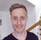cancel
Showing results for
Did you mean:Regular Visitor

## Count the number of occurrences per year

I have 2 table, first one is the payment of each invoice, and another one is member database.

Payment Table

 [user_code] [payment_date] [payment_type] user_A 1/1/2022 A user_B 1/6/2021 A user_A 1/6/2021 B user_C 1/3/2022 A user_C 1/3/2021 A

Member Table

 [user_code] [active] user_A active user_B active user_C inactive

Need help to find out the following question.

1. add 2 column to Member Tabe to find out the number of times each user enrolled in the course TYPE A/ TYPE B this year

2. Calculate how many users have made payment_type A per year.
3. Calculate how many users have made no payment this year but active.

1 ACCEPTED SOLUTIONSolution Sage

Hi!

I'm assuming you have a relationship between the two tables, with [user_code] as key.

Let's take the questions one by one:

1. Create your columns. Do as below for both (switching out for "B" in the second case). You're counting how many cases there are fore each user_code that has a certain payment_type.

``````enrolledA = CALCULATE(
counta(PaymentTable[[user_code]]]),
PaymentTable[[user_code]]] = earlier(MemberTable[[user_code]]]),
PaymentTable[[payment_type]]] = "A")``````

2. Using your new column, create a measure that calculated the number of payments:

``````UsersPaidA = CALCULATE(COUNT(MemberTable[[user_code]]]),
MemberTable[enrolledA]>0)``````

Using this measure along with a date in any visual will get you the number of payments per year.

3. I did this with two measured. First, the number of payments this year:

``````PaymentsThisYear = calculate(Count(PaymentTable[[payment_date]]]),
YEAR(PaymentTable[[payment_date]]]) = year(TODAY()))``````

Then, I used this measure to see how many users that have 0 payments but still an active status.

``````NoPaymentsButActive = CALCULATE(COUNT(MemberTable[[user_code]]]),
FILTER(MemberTable,[PaymentsThisYear] = 0),
MemberTable[[active]]]="active")``````

That should be it. Hope it helps!

2 REPLIES 2Super User

Hey @Mark022 ,

I have tried to implement your ask. See if this is what you are after.

Attaching the pbix file here.Solution Sage

Hi!

I'm assuming you have a relationship between the two tables, with [user_code] as key.

Let's take the questions one by one:

1. Create your columns. Do as below for both (switching out for "B" in the second case). You're counting how many cases there are fore each user_code that has a certain payment_type.

``````enrolledA = CALCULATE(
counta(PaymentTable[[user_code]]]),
PaymentTable[[user_code]]] = earlier(MemberTable[[user_code]]]),
PaymentTable[[payment_type]]] = "A")``````

2. Using your new column, create a measure that calculated the number of payments:

``````UsersPaidA = CALCULATE(COUNT(MemberTable[[user_code]]]),
MemberTable[enrolledA]>0)``````

Using this measure along with a date in any visual will get you the number of payments per year.

3. I did this with two measured. First, the number of payments this year:

``````PaymentsThisYear = calculate(Count(PaymentTable[[payment_date]]]),
YEAR(PaymentTable[[payment_date]]]) = year(TODAY()))``````

Then, I used this measure to see how many users that have 0 payments but still an active status.

``````NoPaymentsButActive = CALCULATE(COUNT(MemberTable[[user_code]]]),
FILTER(MemberTable,[PaymentsThisYear] = 0),
MemberTable[[active]]]="active")``````

That should be it. Hope it helps!Announcements#### Exclusive opportunity for Women!

Join us for a free, hands-on Microsoft workshop led by women trainers for women where you will learn how to build a Dashboard in a Day!#### Power Platform Conference-Power BI and Fabric Sessions

Join us Oct 1 - 6 in Las Vegas for the Microsoft Power Platform Conference.Top Solution Authors
Top Kudoed Authors
Users online (1,660)